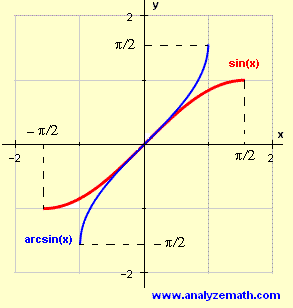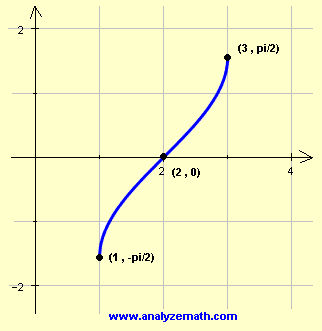# Graphing arcsin(x) functions

A step by step tutorial on graphing and sketching arcsin functions where also the domain and range of these functions and other properties are discussed.

## Graph, Domain and Range of arcsin(x)

In what follows, arcsin(x) is the inverse function of f(x) = sin(x) for - ?/2 ? x ? ?/2.
The domain of y = arcsin(x) is the range of f(x) = sin(x) for -?/2 ? x ? ?/2 and is given by the interval [-1 , 1]. The range of arcsin(x) is the domain of f which is given by the interval [-?/2 , ?/2]. The graph, domain and range of both f(x) = sin(x) for -?/2 ? x ? ?/2 and arcsin(x) are shown below.A table of values of arcsin(x) can made as follows:
 x -1 0 1 y = arcsin(x) -π/2 0 π/2

Note that there are 3 key points that may be used to graph arcsin(x). These points are: (-1,-?/2) , (0,0) and (1,?/2).

### Example 1

Find the domain and range of y = arcsin(x - 2) and graph it.

Solution to Example 1
The graph of y = arcsin(x - 2) will be that of arcsin(x) shifted 2 units to the right. The domain is found by stating that -1 ? x - 2 ? 1. Solve the double inequality to find the domain:
1 ? x ? 3
The 3 key points of arcsin(x) can also be used in this situation as follows:

 x - 2 -1 0 1 y = arcsin(x-2) -π/2 0 π/2 x 1 2 3

The value of x is calculated from the value of x - 2. For example when x - 2 = -1, solve for x to find x = 1 and so on.
The domain is given by the interval [1,3] and the range is given by the interval [-?/2,?/2]
The three points will now be used to graph y = arcsin(x - 2).### Example 2

Find the domain and range of y = 2 arcsin(x + 1) and graph it.

Solution to Example 2
We use the 3 key points in the table as follows, then find the value 2 arcsin(x + 1) and x.

 x + 1 -1 0 1 arcsin(x+1) -π/2 0 π/2 y = 2 arcsin(x+1) -π 0 π x -2 -1 0

domain = [-2,0] , range = [- ? , ?]The graph is that of arcsin(x) shifted one unit to the left and stretched vertically by a factor of 2.

### Example 3

Find the domain and range of y = - arcsin(x - 1) and graph it.

Solution to Example 3
We use the 3 key points in the table as follows, then find the value - arcsin(x - 1) and x.

 x - 1 -1 0 1 arcsin(x-1) -π/2 0 π/2 y = - arcsin(x-1) π/2 0 -π/2 x 0 1 2

domain = [0 , 2] , range = [- ?/2 , ?/2]The graph is that of arcsin(x) shifted one unit to the right and reflected on the x axis.

## More References and Links to Graphing

Graphing Functions